模式匹配_模式匹配算法 - CSDN

• ## 模式匹配算法

千次阅读 2017-09-18 16:24:50
串的模式匹配设有两个字符串S和T，设S 为主串，设T为子串。在主串S中查找与模式T相匹配的子串，如果匹配成功，确定相匹配的子串中的第一个字符在主串S中出现的位置。 一、 BF算法： 1. 指针i和j 分别指示主串S 和...
串的模式匹配设有两个字符串S和T，设S 为主串，设T为子串。在主串S中查找与模式T相匹配的子串，如果匹配成功，确定相匹配的子串中的第一个字符在主串S中出现的位置。
一、  BF算法：
1.  指针i和j 分别指示主串S 和模式串T 中当前待比较的字符位置，i 和j初始值为1；
2.  取k来代替i从当前位置开始与j相匹配，此时的i用来记录它本来的值 而k是要随着j的变化而变化的
3.  遇到不匹配的情况时，从i的下一位开始与j进行再一次的比较
这种方法简单易理解易实现 但是过于冗余 复杂度较高

#include <iostream>
#include<cstdio>
#include<cstring>
using namespace std;

int main()//BF算法，从主串的第一位开始 主串与模式串比较，不匹配时主串的指针要回去从下一位开始匹配
{
int i,j,k,ans=-1;
char S,T;
scanf("%s%s",&S,&T);
int lens,lent;
lens=strlen(S);//主串的长度
lent=strlen(T);//子串的长度
for(int i=0;i<lens;++i){//从下一位重新开始匹配
for(j=0,k=i;j<lent&&k<lens;++j){
if(S[k]==T[j])
++k;
else//也即 此时不匹配跳出匹配过程
break;
}
if(j==lent){
ans=i;
break;
}
}
printf("%d\n",ans);//输出开始位置 -1为未匹配成功
return 0;//此方法虽然能够找到答案，但是时间复杂度很高 重复的过程很多即很慢
}

二、KMP
上面的BF算法 不如说 在S串中找到位置k 从k开始与T匹配 之前 需要遍历S从0到k 而kmp算法所做的工作就是让我们不必从0到k一步一步都遍历一遍 而是跳着匹配 这就要求我们在遇到不匹配的情况时需要知道下一步跳到哪里开始重新匹配 也就是kmp中的next数组了。例如S 串为：ababcabcacbab T串为：abaca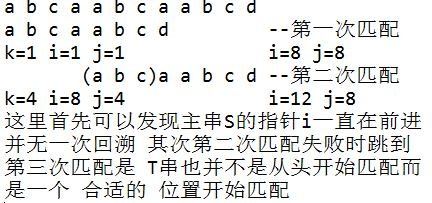而所谓的 合适的 位置是如何得来的呢
现在假设应与T串中的第k个字符继续比较 例如上图第二次匹配，则T串的从1到k-1个字符应该是与S串的从（此时的i是此次匹配开始值i=8）i-k+1到i-1个字符相匹配的，而在第一次匹配过程中可以发现，S串的从（i=8, j=8）i-k+1到i-1个字符是与T串中j-k+1到j-1个字符是匹配的，所以第二次开始时跳到k=4是因为
T串的从1到k-1个字符 和T串的从j-k+1到j-1个字符相匹配（j=8）
也就是说kmp基于的是模式串即T串本身的一种 轮回？现在就是在基于模式串来求next数组，以发生不匹配时可以跳到合适的位置
当j=1时next[j]=0
next[j]=Max{ k| 1< k < j && T1…..Tk=Tj-k+1……Tj-1}即寻找最大的轮回
当k=1时 也就是没有所谓的轮回时next[j]=1
在匹配过程中 若Si==Tj 或者j==0则 i j 都自增1 否则 j 跳到next[j]位置
然后就是求next数组了 由next数组的定义可知next=0
假设next[j]=k 这表示在T串中 T1…..Tk-1 = Tj-k+1……Tj-1
讨论next[j+1]的值 当Tk==Tj 时 显然 next[j+1]=k+1;不等时 仔细想一下 这个过程是不是刚好是串的模式匹配过程 不过这里主串和模式串都是T串 不等时把身为模式串的T串右移 右移到哪呢 到next[k]啊(这里可以拿纸笔自己演算) 默认的存在 k < j 所以此时next[k]是已知条件 这时若Tj==Tnext[k] 则 next[j+1]=next[k]+1 不等就继续找啊 类似dfs一层一层往下找 直到Tj!=T1 此时next[j+1]=1（最好的理解方式就是拿纸笔从头到尾自己演算一遍 不省略任何一个步骤）
ok 这里说明一下 由于本人偷懒 简陋的代码里字符串是从0开始存的 next=-1 中心思想是没变的

#include <iostream>
#include<cstdio>
#include<cstring>
using namespace std;
int main()
{
int next;
char S,T;
scanf("%s%s",&S,&T);
int lens=strlen(S);
int lent=strlen(T);
int i=0,j=-1,ans=-1;
next[i]=j;
while(i<lent){
if(j==-1||T[i]==T[j]){
++i;
++j;
//if(T[i]!=T[j])
next[i]=j;
//else
//next[i]=next[j];
}
else
j=next[j];
}
i=0,j=0;
while(i<lens){
if(j==-1||S[i]==T[j]){
++i;
++j;
}
else
j=next[j];
if(j==lent){
ans=i-j;
break;
}
}
printf("%d\n",ans);//此答案为从0开始计数。。。
return 0;
}

最后讲一下求nextval数组 可以发现 代码中注释掉的部分 添上就是求nextval数组了
这里发现一件很有意思的事情 之前求next数组时 next[j]=k 求next[j+1]值时第一种情况就是Tj==Tk 现在讨论这种情况下 主串S和模式串T匹配过程中刚好到 j 这个位置失配了 此时 由next[j]=k 就要跳到 k 这个位置进行比较了 可是Tj==Tk啊 不用比较就知道不匹配啊 可是前面求的时候就这样求的 错了吗 没啊 前面没错 这里也没错 可是怎么就不一样了呢 因为前面是根据这两个求的是next[j+1]的值啊 讨论的是j+1不匹配的时候跳到哪里 这里是j不匹配跳到哪里 ok 清楚就行
nextval数组处理的就是上面这种情况 减去无谓的匹配 这也就有了代码中的注释部分
最后 此篇文章借鉴于严蔚敏等主编的数据结构（C语言版）课本 有错欢迎指出 谢谢

展开全文kmp算法详解
• 一般的字符串模式匹配算法是类似下面的逐次匹配，举例说明如下 主串s=ababcabcacbab 从串t=abcac 一般匹配方法如下图所示 代码如下 int index(string s,string t) {  int i=0,j=0;  int l1=s.length();  ...
一般的字符串模式匹配算法是类似下面的逐次匹配，举例说明如下

主串s=ababcabcacbab

从串t=abcac

一般匹配方法如下图所示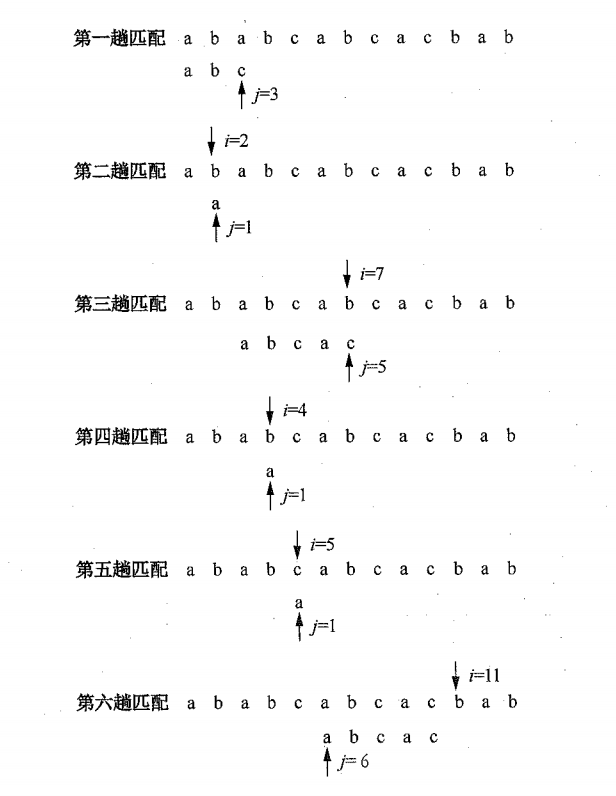代码如下

int index(string s,string t)
{
int i=0,j=0;
int l1=s.length();
int l2=t.length();
while(i<=l1-1&&j<=l2-1)
{
if(s[i]==t[j])
{
++i;
++j;
}
else
{
if(j==0)
{
++i;
}
else
{
i=i-j+2;
j=0;
}

}
//cout<<"i="<<i<<"j="<<j<<endl;
}
if(j>l2-1) return i-l2;
else return 0;
}

这样算下来的时间复杂度是O（l1*l2），因为每次只要中间发生字符串s[i]和t[j]不相等，这种算法会把字符串t的索引置为0，而主串s也是从之前开始匹配的i加一个1，其实我们可以发现，中间有些比较是不必要的，比如从第三趟比较就可以看出主串中第4，5,8个字符串是‘b’,'c','a'，（对应模式串中的第2,3,4个字符）。而模式串t中第一个字符是a，所以其实不需要和这几个字符相比较了，只需要将模式向右滑动3个字符即可。这样的匹配过程中，实际上主串i没有回溯，只是模式串的j在变化，就降低了时间复杂度为O（l1+l2）,这也就是kmp算法。

kmp算法每趟比较过程让模式串向后滑动一个合适的位置，这个合适的位置我们可以算出来，一般称这个位置叫next表。

先写出next表的定义，接下来再解释为什么这样定义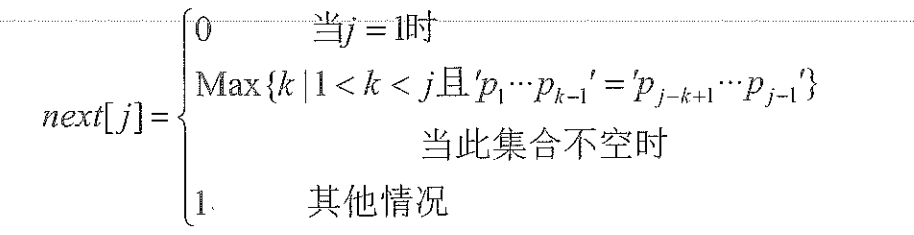结合这个图来解释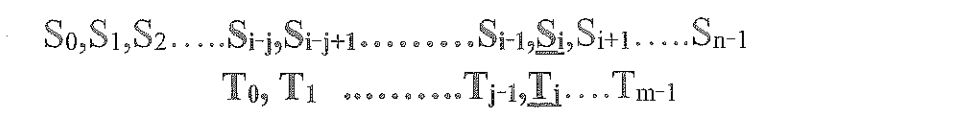先说一下，上面两个图中的S0和T0分别代表的是两个穿的长度，真正的字符串都从下标1开始。

1）当j=1时，也就是说模式串的第一个字符就与当前位置s对应的字符不同，此时主串的下标应该+1，再和模式串第一个字符进行比较；

2）当两个串匹配到此处时，前j-1个字符都是匹配的，到了第j个字符就不同了，此时需要把字符串t向右移动max{k}个位置。

这个k应该满足1<k<j并且p1...pk-1=pj-k+1...pj-1.k的值可能有多个，为了不使向右移动丢失可能的匹配，选择最大的一个k，也就是max{k}，其最大值为j-1；

3）除了上面两种情况，发生匹配时，主串指针i不回溯，在最坏情况下，模式串从第1个字符开始与主串第i个字符比较，以便不丢失可能的匹配。

上面讲解的next函数表的定义，然后下面是求next函数表的代码以及实现kmp算法。篇幅有点长，转到下一篇讲。


展开全文kmp算法
• 模式匹配 Scala 中的模式匹配类似于java中的 switch 语法, 但是更加强大 模式匹配语法中, 使用 match关键字声明, 每个分支采用 case 关键字进行声明, 当需要匹配是,会在第一个case 分支开始,如果匹配成功,那么执行...
模式匹配
Scala 中的模式匹配类似于java中的  switch  语法,  但是更加强大
模式匹配语法中,  使用 match关键字声明, 每个分支采用 case  关键字进行声明, 当需要匹配是,会在第一个case 分支开始,如果匹配成功,那么执行对应的逻辑代码,如果匹配不成功,继续执行下一个分支进行判断.
如果所有  case  都不匹配, 那么会执行 case _ 分支, 类似于  java  中的 default语句
1.1基本应用
ex1:  输入1,2,3  会打印对应的颜色
object Color {

def main(args: Array[String]): Unit = {
//从键盘获取数字
i match {
case 1 => println("red")
case 2=> println("green")
case 3=> println("yellow")
case _=> println("您的输入有误")
}
}

}


说明:

如果所有case都不匹配，那么会执行case _ 分支，类似于 Java 中default语句
如果所有case都不匹配，又没有写case _ 分支，那么会抛出异常
每个case中，不用break语句，自动中断case
可以在match中使用其它类型(任意类型)，而不仅仅是字符
=> 等价于 java swtich 的 :
=>后面的代码块到下一个 case， 是作为一个整体执行，可以使用{} 扩起来，也可以不括

ex2 :  加减乘除
object MatchDemo2 {
def main(args: Array[String]): Unit = {
var a1 = 10
var a2 = 20

var operChar = "*"
val res = operChar match {
case "+" => a1 + a2
case "-" => a1 - a2
case "*" => a1 * a2
case "/" => a1 / a2
case _ => "你的运算符负不正确"
}
println("res = " + res)
}
}

1.2 守卫
想要表达某个范围的数据, 就需要在匹配模式匹配中增加条件"守卫"
object MatchDemo3 {
def main(args: Array[String]): Unit = {
for (ch <- "+-3&%") {
var digit = 0
val sign = ch match {
case '+' => 1
case '-' => -1
case _ if Character.isDigit(ch) => digit = Character.digit(ch, 10)
case _ => 0
}
println("ch = " + ch)
println("sign = " + sign)
println("digit = " + digit)
println("---------")
}
}
}

1.3 变量和常量
如果在case关键字后跟变量名,那么 match 前表达式的值会赋值给那个变量
object MatchVar {

def main(args: Array[String]): Unit = {
var ch = 0

'z' match {
case 'a' => println("a...")
case 'b' => println("b...")
case 'c' => println("c...")
case ch => println("ch=" + ch)
}
}
}


注意

如果 case ch 匹配成功了, 后面即使跟上case _ 也没有用
可以把case _ 看成 case 变量名 的特例 ,  把_看成一个变量来对待

但是:

按照约定, Scala 期望模式变量名都以小写字母开头, 而常量名则是大写字母(只要首字母大写也可以)。

如果你使用大写字母的名称，Scala 将会在作用域范围内查找常量。
但是，如果使用的是非大写字母的名称，它将只假设其是一个模式变量—-在作用域范围内任何具有相同非大写字母的名称都将会被忽略。
在下面的代码中，我们定义了一个和字段具有相同名称的模式变量, 但是这段代码将不会给出我们想要的结果—–模式变量隐藏了（Sample 类的）max 字段。
object PatternTest {
def main(args: Array[String]): Unit = {
val sample = new Sample
sample.process(1000)
}
}

class Sample {
val max = 10000
def process(input: Int): Unit = {

input match {
case max => println(s"You matched max")
}
}
}


说明:

你会发现 scala 把 max推断为模式变量, 而不是模式常量.

解决办法:

办法1: 明确指定作用域:this.max

办法2: 使用反引号类给 scala 提示.
 case max => .....


办法3: 把max变成大写: MAX

1.4 模式匹配
object MatchType {

def main(args: Array[String]): Unit = {

val list = List(1, 3.2, "sa",true)

var result = list match {
case a: List[Int] => println("...Int类型...")
case b : List[String] => println("...string...")
case c :List[Double] =>println("...double...")
case d:List[Boolean] => println("...boolean...")

}
println(result)
}
}


说明:

在进行类型匹配时，编译器会预先检测是否有可能的匹配，如果没有则报错
如果类型匹配成功之后会把s的值赋值给case后面跟着的变量, 而不需要做类型的转换.
对数组来说提供数组元素中的类型是必要的,因为数组的底层类型确实是确定的.比如:Array[Int]这里的Int是必须的
但是对集合类型比如 Map, 提供泛型类型是无用的. 因为 Java 的"泛型擦除". Map[Int, String]和Map[Int, Int] 在匹配的时候没有区别. 所以应该使用通用的泛型:Map[_, _]

import scala.io.StdIn

object MatchType {
def main(args: Array[String]): Unit = {
var s: Any = Map("a" -> 11, "b" -> 22)

var result = s match {
case d: Map[_, _] => "匹配到的是Map"
case _ => "啥都没有匹配到"

}
println(result)
}
}

1.5 匹配数组
object PatternDemo5 {
def main(args: Array[String]): Unit = {
// Array(1,2,3) == Array(1,2,3)
var arr1 = Array(1, 2, 3, 5, 6)

arr1 match {
//匹配三个元素,只能是三个元素,且为123
//            case Array(1, 2, 3) => println("Array(1,2,3)")

//匹配四个元素,且前两个元素为1,2
//            case Array(1, 2, _, _) => println("Array(1,2,_,_)")

//匹配四个元素,第三个元素用变量接收
//            case Array(1, 2, a, _) => println("Array(1,2,_,_)  " + a)

//匹配前两个元素为1,2,后面是什么都行
//            case Array(1, 2, _*) => println("Array(1,2, _*)  ")

//匹配前两个为1,2,  后面为一个数组
// 在未来 @ 会变成 :
//            case Array(1, 2, rest@_*) => println("Array(1,2, rest@_*)  " + rest.mkString(", "))
case Array(_, _, rest@_*) => println("Array(_,_, rest@_*)  " + rest.mkString(", "))
case _ => println("no ")

}

}
}

1.6 匹配列表
def main(args: Array[String]): Unit = {
val arr: List[Int] = List(1, 2, 3, 5, 6)
val res = arr match {
//case List(1, 2) => "List(1, 2)"
//case List(1, _*) => "匹配以 1 开头的列表"
//case 1 :: 2 :: 3 :: Nil => "匹配List(1,2,3)"
case 1 :: abc => println(abc); "匹配以 1 开头的列表"
case _ => "啥也可没有匹配到"
}
println(res)
}

1.7 匹配元组
object MatchTouple {

def main(args: Array[String]): Unit = {

//        val tup1:(Int , String)= (1,"niefeng")
val tup2:(Int , String , String)= (2,"bujingyun","chuchu")

tup2 match {
case (a,b,c) => println(s"a=$a,b=$b,c=$c") } } }  1.8 对象匹配(提取器) 对象匹配，什么才算是匹配呢？，规则如下: case中对象的unapply方法(提取器)返回some集合则为匹配成功 返回None集合则为匹配失败 备注: Some和None 都是Option的子类型 从2.11.1 开始, scala 为了性能上的优势, 放松了对unapply返回的值的类型的限制(不必必须是 Option). 返回的类型只要具有以下方法就可以: def isEmpty: Boolean 返回值: true用来标记匹配成功, false匹配失败 def get: T 返回值: 表示提取到的值. 其实Some和None 均包含上面的两个方法. 另外一种情况: 如果unapply返回一个Boolean值, 则只表示匹配成功或者失败. 如果是 true则表示匹配成功, 但是不会提取到任意的值. 不过, 大部分情况下, 我们还是把要提取的数据封装到Some中返回. 1. 提取单个对象 object MatchObj { def main(args: Array[String]): Unit = { val num = 100.0 num match { case Square(z) => println("z =" +z) } } } object Square{ def apply(a:Double): Double = a * a def unapply(arg: Double): Option[Double] = Some(math.sqrt(arg)) } result: 10.0  执行流程说明: 在 case匹配的过程中, 如果碰到这种(伴生对象(参数))的形式的时, 就会调用这个伴生对象的unapply方法, 来提前对象. 调用unapply的时候, 会把前面的匹配表达式(本例中的num)传递给unapply 如果unapply返回的是Some, 则表示匹配成功. 并unapply的返回值赋值给伴生对象(参数)中的参数(本例中的z), z其实就是提取到的对象 如果unapply返回的None, 则表示匹配失败. 继续下一个case的匹配… 2. 提取多个对象 object MatchObjs { def main(args: Array[String]): Unit = { val s = "yangguo,xiaolongnv" val res = s match { case Names(one,two) => println(s"成功! one =$one,two =$two") case _ => println("匹配失败") } } } object Names{ def unapplySeq(str:String)={ if (str.contains(",")) Some(str.split(",")) else None } }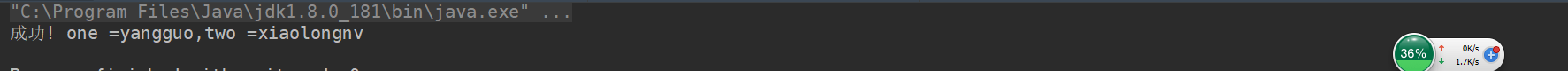执行过程说明: case Names(one, two), 这里有 32个需要提取的结果, 所以会调用伴生对象.unapplySeq方法. 如果这个方法返回的是Some, 并且 Some中的序列有 2个值, 则匹配成功. 否则就匹配失败. 1.9 变量声明中的模式 在声明变量的时候,也可以使用模式匹配的方式. (在其他语言中叫做解构) object VarMatch { def main(args: Array[String]): Unit = { var (a: Int, b: Int) = (10, 20) println(s"a =$a, b=$b") val (aa, bb) = BigInt(10) /% 3 println(s"aa =$aa, bb = $bb") val arr = Array(100, 200, 300, 400) val Array(c, _, d, _*) = arr // println(s"c =$c, d = $d") } }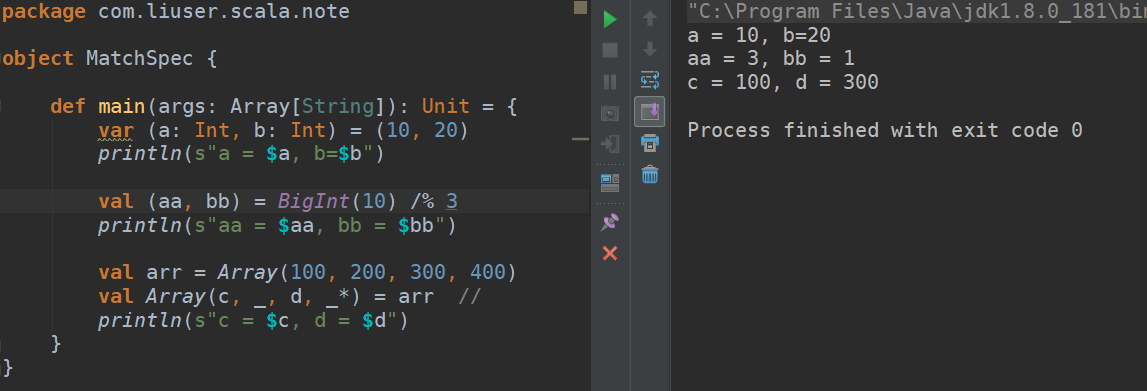1.10 for表达式中的模式 def main(args: Array[String]): Unit = { val map = Map("a" -> 1, "b" -> 2, "c" -> 3, "d" -> 2) // 直接将Map中的K-V遍历出来 for ((k, v) <- map) { println(s"k =$k, v = $v") } println("--------------") // 只遍历 v = 2的 k-v for((k, 2) <- map) { println(s"k =$k")
}
println("--------------")
// 也可以使用 守卫: 遍历v > 1的
for ((k, v) <- map if v > 1){
println(s"k = $k, v =$v")
}
}

1.11 样例类
案例1
object CaseClassDemo1 {
def main(args: Array[String]): Unit = {
val arr = Array(Dollar(1000), Currency(10000, "RMB"))

for (ele <- arr) {
val res = ele match {
case Dollar(v) => "\$" + v
case Currency(v, u) => v + u
case _ => ""
}
println(res)
}
}
}

// 一个抽象类
abstract class Amount {}

// Dollar: 样例类 继承自 Amount
case class Dollar(value: Double) extends Amount {}

// Currency: 样例类
case class Currency(value: Double, unit: String) {}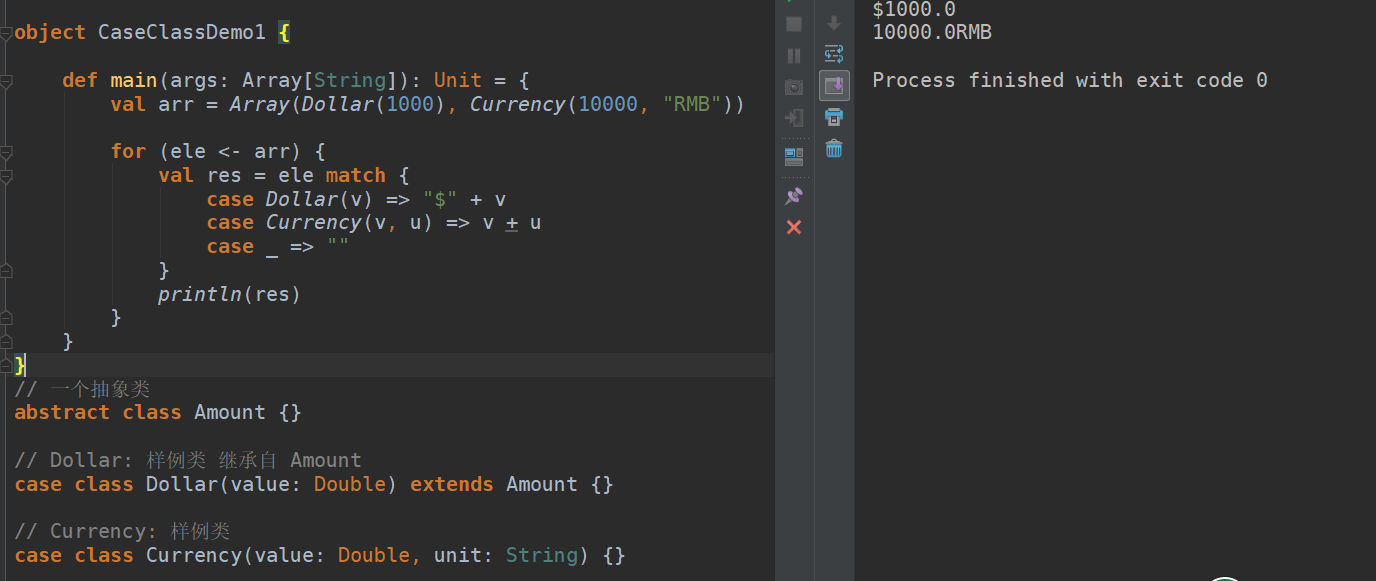案例2
copy会创建一个与现有对象值相同的新对象，并可以通过带名参数来修改某些属性。
object CaseClassDemo2 {
def main(args: Array[String]): Unit = {
val amt1: Currency = Currency(122.2, "美元")
// 复制一个与 amt1 完全相同的对象
val amt2: Currency = amt1.copy()
val amt3: Currency = amt1.copy(value = 222.2)
val amt4: Currency = amt1.copy(unit = "英镑")
println(amt1)
println(amt2)
println(amt3)
println(amt4)
}
}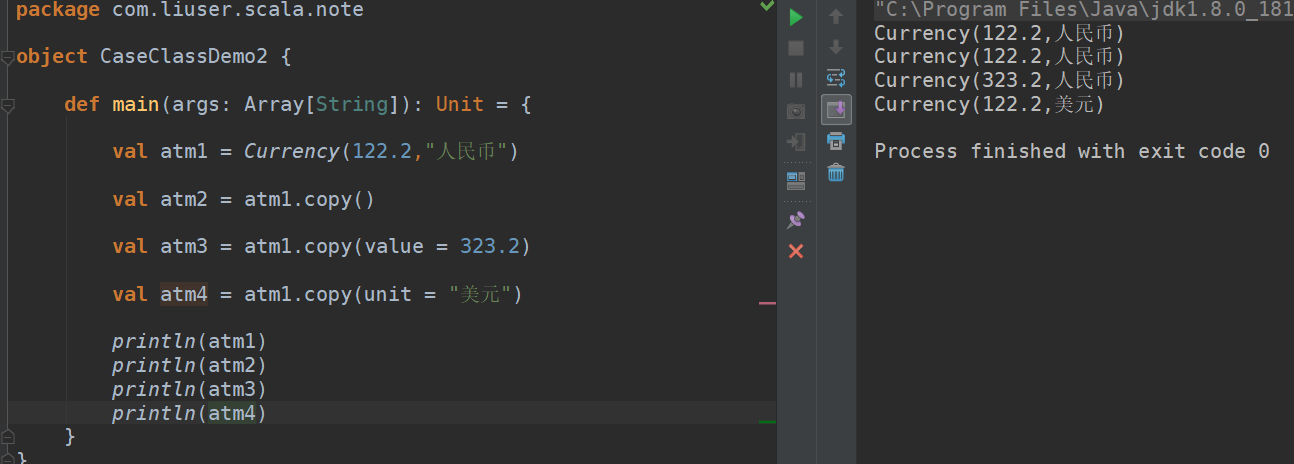案例3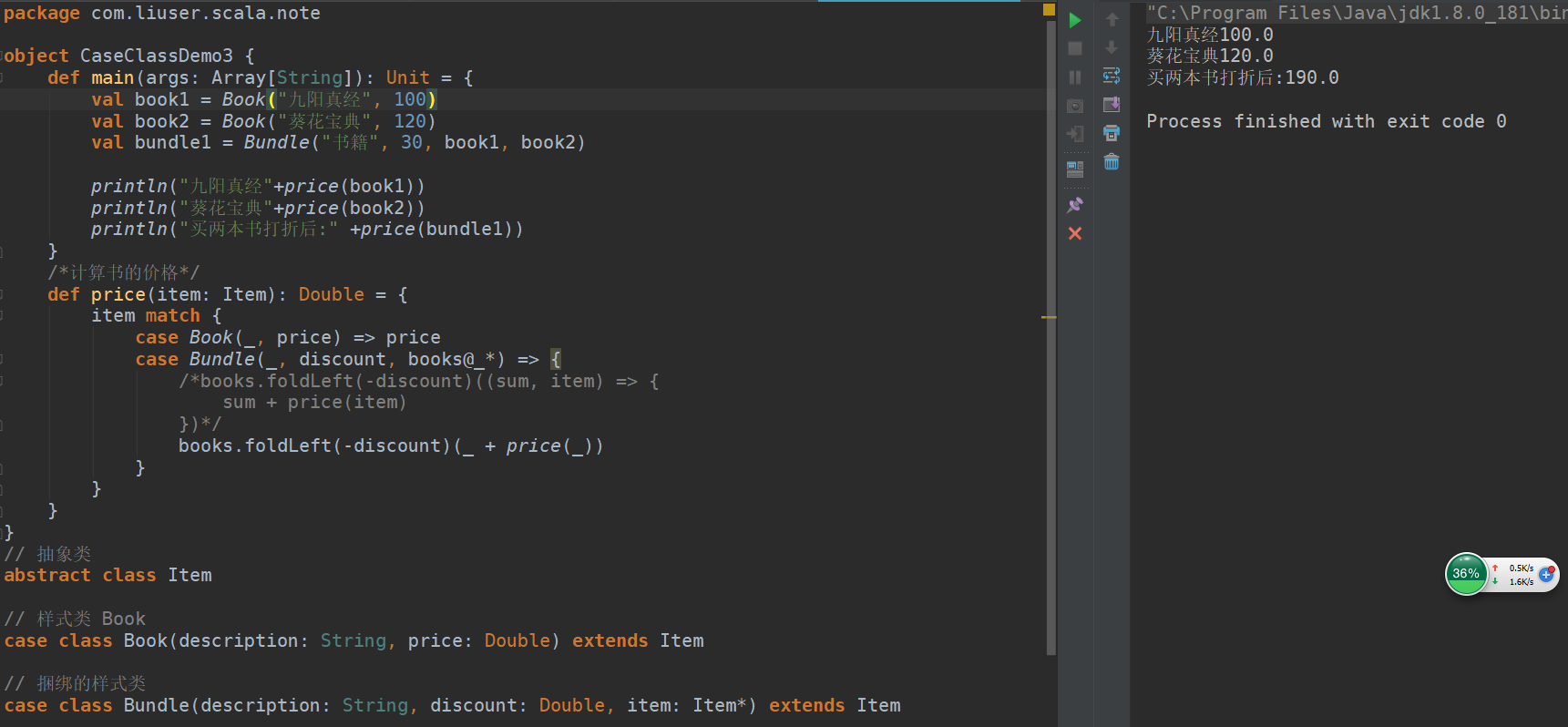1.12 case语句中的中置表示法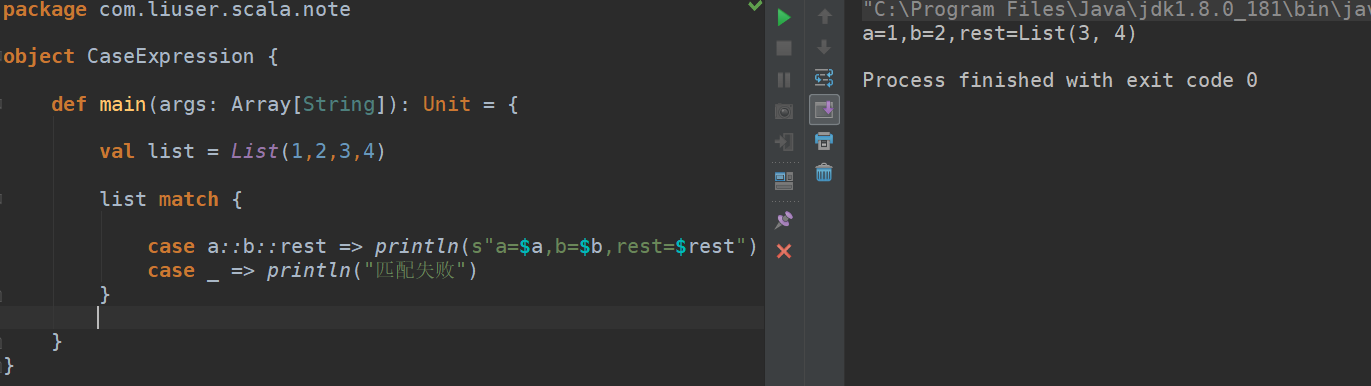)
1.13 匹配嵌套结构
object MatchNest {
def main(args: Array[String]): Unit = {
val book1 = Book("九阳真经", 100)
val book2 = Book("葵花宝典", 120)
val bundle1 = Bundle("书籍", 20, book1, book2)

println(price2(book1))
println(price2(book2))
println(price2(bundle1))

}

/**
* 计算打包的销售的书的价格
*
* 方式1:
*/
def price(item: Item): Double = {
val money = item match {
case Book(_, price) => price
case Bundle(_, discount, items@_*) => {
var sum = -discount
for (elem <- items) {
sum += price(elem)
}
sum
}
case _ => 0
}
money
}
// 方式2
def price2(item: Item): Double = {
item match {
case Book(_, price) => price
case Bundle(_, discount, items@_*) => {
// 不用循环
// 把每本书的价格映射到新的序列中, 然后再求和
items.map(price2).sum - discount
}
case _ => 0
}
}
}

// 抽象类
abstract class Item

// 样式类 Book
case class Book(description: String, price: Double) extends Item

// 捆绑的样式类
case class Bundle(description: String, discount: Double, item: Item*) extends Item

1.4 密封类
package com.liuser.scala.note

object MatchSeal {

def main(args: Array[String]): Unit = {
val amounts = Array(Dollar1(100),Dollar1(200), new Currency1(100,"rmb"))

for (elem <- amounts) {
elem match {
case Dollar1(v) => println("values=" + v)
case Currency1(v,u)=>println("v=" +v ,"u="+u)
case _ => println("匹配失败")
}
}

}
}
//密封类
sealed abstract class Amount1{}

case class Dollar1(value:Double) extends Amount1{}

case class Currency1(value:Double,unit : String) extends Amount1{}

1.5 模拟枚举类
object EnumDemo {
def main(args: Array[String]): Unit = {
val seasons = Array(Spring, Summer, Autumn, Winter)
for (season <- seasons) {
val msg = season match {
case Spring => "春天"
case Summer => "夏天"
case Autumn => "秋天"
case Winter => "冬天"
case _ => "每在地球上"
}
println(msg)
}
}
}

sealed abstract class Season

case object Spring extends Season

case object Autumn extends Season

case object Summer extends Season

case object Winter extends Season



展开全文scala 模式匹配
• 一、朴素模式匹配朴素模式匹配算法就是遍历主串，然后把待匹配字符串与子串进行比对，先把待匹配子串的第一个字母与主串进行匹配，若匹配成功，则两串的坐标依次 ++，匹配不成功时，主串坐标返回到开始匹配时的坐标...
一、朴素模式匹配朴素模式匹配算法就是遍历主串，然后把待匹配字符串与子串进行比对，先把待匹配子串的第一个字母与主串进行匹配，若匹配成功，则两串的坐标依次 ++，匹配不成功时，主串坐标返回到开始匹配时的坐标，待匹配串坐标清零，若待匹配坐标等于待匹配子串长度，则证明匹配成功， 返回匹配完毕主串的第一个坐标，否则返回-1假设主串的长度为N，待匹配串的长度为M，因为需要遍历主串，每次匹配的长度都小于等于M，所以  它的时间复杂度是O(M*N)的	public static int puSu(String str1, String match1) {
int length1 = str1.length();
int length2 = match1.length();
//i，j，k分别表示的是主串下标、匹配串下标、记录下次循环主串开始的位置
int i = 0;
int j = 0;
int k = 0;
char [] str = str1.toCharArray();
char [] match = match1.toCharArray();
while(i < length1 && j < length2) {
if(str[i] == match[j]) {
i++;
j++;
}else {
k++;
j = 0;
i = k;
}
}
if(j == length2) {
return k;
}else {
return -1;
}
}
public static void main(String[] args) {
String str = "asdfghg";
String m1 = "sd";
System.out.println(puSu(str, m1));
System.out.println(puSu(str, m2));
}二、KMP模式匹配算法KMP模式匹配算法因为朴素匹配的时间复杂复杂度为O(M*N)，因此我们寻求一个时间复杂度较小的模式匹配算法，KMP，一般用来表示克努斯-莫里斯-普拉特算法（Knuth-Morris-Pratt），是在一个“主文本字符串” Str内查找一个“词” Match 的出现，通过观察发现，在不匹配发生的时候这个词自身包含足够的信息来确定下一个匹配将在哪里开始， 以此避免对以前匹配过的字符重新检查。KMP模式匹配算法主要解决的是传统朴素模式匹配算法，当主串从i开始进行匹配，当匹配到j位置时，发现不匹配，主串跳回i+1位置，匹配串跳回0位置，这就导致匹配的效率很低，时间复杂度很高。KMP则当到j位置不匹配时，主串不动，匹配串先通过计算从当前位置的前缀子串和后缀子串的最大匹配串的长度， KMP算法的精髓就是在于求nextArray的过程，这个数组的作用就是把待匹配字符串从头开始向右滑动和主串进行匹配（每次滑动的大小就是nextArray[i]存储的值的大小）从而省去了match字符串返回到终点和主串返回到i+1位置 上的过程。 逻辑思路： ①求nextArray数组的值。我们在进行KMP模式匹配的时候，因为每次匹配失败，match字符串都要向右滑动nextArray[j-i]个长度的值，所以，第一步就是要求得nextArray数组的值。如何求nextArray的值？首先我们来看nextArray数组的定义：当主串从i位置开始匹配，到j位置失败的时候，match指针现在的位置是在j-i位置上的，我们得到一个必须一match开头的前缀字符串，必须以match[j-i-1]结尾的后缀字符串，这两个字符串的最大匹配长度就是nextArray[j-i]的值。举个例子：假设match="aaaaabb",这个字符串，匹配失败的时候j-i的值为6，则match[j-i]="b",所以它之前的字符串为 "aaaaab",其前缀字符串为match[1,2,3,4,5]="aaaab",后缀字符为match[0,1,2,3,4]="aaaaa"所以它的最大匹配字符串为"aaaa",长度为4，所以，nextArray[j-i]的值为4.②当主串从i位置开始匹配的时候，匹配到j位置发现不匹配。如果，此时nextArray的值为-1也就是没有找到滑动的字符串 的时候，主串的位置++，否则，把match的字符串的从头向右滑动nextArray[j-i]个字符，让match[k],k的值为 nextArray[j-i]的值+1，与str[j]的值进行匹配，直至str在某一位置把match匹配完，则证明str中有match 返回的位置为si-mi的值，如果match滑到最后也没有匹配出来，则证明该主串中没有match，返回-1，整个过程结束。public static int getIndexOf(String s, String m) {
if(s == null || m == null || m.length() < 1 || s.length() < m.length()) {
return -1;
}
char [] ss = s.toCharArray();
char [] ms = m.toCharArray();
int si = 0;
int mi = 0;
int [] nextArray  = getNextArray(ms);
while(si < s.length() && mi < m.length()) {
if(ss[si] == ms[mi]) {
si++;
mi++;
}else if(nextArray[mi] == -1) {
si++;
}else {
mi = nextArray[mi];
}
}

return mi == m.length() ? si - mi : -1;

}

//求nextArray的过程
public static int[] getNextArray(char[] ms) {
//当待匹配串不匹配时已匹配串的的长度+1等于1时，由定义可知nextArray==-1
if(ms.length == 1) {
return new int [] {-1};
}
int [] nextArray = new int [ms.length];
//由定义可知，nextArray的第一个值和第二个值为-1和0
nextArray = -1;
nextArray = 0;
//匹配失败的位置
int pos = 2;
//因为是从头向后进行匹配，所以在算nextArray[j-1]的值得时候nextArray[j-i-1]的值就已经知道了
//cn代表的值就是最大匹配长度，他的含义是已匹配字符串的前缀字符串的下一个字符串，
//match[cn]和match[j-i-1]进行比较,若相等，则nextArray[j-i] = nextArray[j-i-1]+1
//若不相等，则看match[cn]这个字符的最长前缀和后缀匹配情况，也就是求nextArray[cn],
//求这个数组的长度和上一步一样，看nextArray[cn-1]的值，和两个前缀字符串假设两个区域是n和m，n的下一个
//字符串的值为x，则判断x的值和match[cn-1]的值是否相等，若相等，则nextArray[j-i] = nextArray[cn]+1
//不相等则继续进行判断，直至match[cn] <= 0时证明该段字符串的最长匹配值为0，即nextArray[j-i] = 0
int cn = 0;
while(pos < ms.length) {
if(ms[pos] == ms[cn]) {
nextArray[pos++] = ++cn;
}else if(cn > 0) {
cn = nextArray[cn];
}else {
nextArray[pos++] = 0;
}
}

return nextArray;
}
public static void main(String[] args) {
String str = "asdfghg";
String m1 = "df";
System.out.println(getIndexOf(str,m1));
System.out.println(getIndexOf(str, m2));
}KMP算法的时间复杂度：匹配的过程会遍历主串，遍历主串的代价是O(N)的，再来看求nextArray这个方法的时间复杂度，主要是两个量，一个是pos这个变量，它的含义就是匹配失败的坐标，所以大小肯定小于M，再来看pos-cn这个变量，也就是滑动的次数，因为破损最大值为M-1，cn最小值为0，所以pos-cn的值还是小于M的，因此它的时间复杂度不会超过2M,也就是O(M)，所有他的时间复杂度为O(N)+O(M)，因为在实际情况中，M的大小要远小于N的，所以，我们可以把它的时间复杂度看成O(N).
展开全文算法 字符串 模式匹配 KMP
• 模式匹配（Java）——烤馍片算法(KMP算法) 模式匹配 模式匹配是数据结构中字符串的一种基本运算。KMP 模式匹配
• Knuth-Morris-Pratt 字符串查找算法，简称为 “KMP算法”，常用于在一个文本串S内查找一个模式串P 的出现位置，这个算法由Donald Knuth、Vaughan Pratt、James H. Morris三人于1977年联合发表，故取这3人的姓氏命名...
• ## 数据结构---串的模式匹配算法介绍

万次阅读 多人点赞 2017-02-22 10:58:37
对于文本程序来说，找出一个子串在文本中的位置是特别重要的，我们称那个子串为模式串（pattern），然后我们称寻找的过程为：模式匹配（string match）。 2、实现算法（1）—朴素字符串匹配算法 原理：从数据结构 算法 KMP 串的模式匹配
• 1.模式匹配(pattern matching)的概念 2. 制造模式匹配的测试串 3. 模式匹配蛮力算法（Brute-Force，也成Naive朴素算法） 3.1 Version 1 3.2 Version 2:（与Version 1的不同在于i，j） 3.3 算法分析 （1）最差...
• 在Expresso中，测试“多行模式” 测试一 注意：这里样例文本中3eeeee后面没有回车，光标就在e的后面。匹配的结果是3eeeee，如上图的Search Results区中所示。 为什么这里不能匹配1abcde和2abc？ 开启多行模式 ...正则表达式 windows php java
• ## 正则基础之——贪婪与非贪婪模式

万次阅读 多人点赞 2011-02-21 01:03:00
1 概述贪婪与非贪婪模式影响的是被量词修饰的子表达式的匹配行为，贪婪模式在整个表达式匹配成功的前提下，尽可能多的匹配，而非贪婪模式在整个表达式匹配成功的前提下，尽可能少的匹配。非贪婪模式只被部分NFA引擎...正则表达式 regex 优化 div
• 1、贪婪匹配和懒惰匹配 影响的是正则表达式的限定符的匹配结果；  在限定符后面加上?，则为懒惰模式；在限定符后面不加?，则为贪婪模式；常用的限定符如下： 如下截图参考：...
• 在前面两篇文章当中已经介绍了python用正则表达式的基本运用，接下来总结一下正则表达式中的贪婪模式和非贪婪模式。 一、概念 首先举个例子： example = "abbbbbbc" pattern = re.compile("ab+") 贪非贪婪模式 正则表达式
• 1 概述 贪婪与非贪婪模式影响的是被量词修饰的子表达式的匹配行为，贪婪模式在整个表达式匹配成功的前提下，尽可能多的匹配，而非贪婪模式在整个表达式匹配成功的前提下，尽可能少的匹配。非贪婪模式只被部分NFA引擎...正则表达式
• 在写这篇之前，我一定要说，我讨厌KMP算法！！！所以我是不会讲解KMP算法的！！！ 好了，开始。 ...创新之处是模式串是从右向左进行比较。...第二步：模式串从不匹配的那个字符开始，从右向左寻找匹配串中不匹配算法 字符串匹配
• ## MySQL之模糊查询

万次阅读 2019-03-25 10:28:29
1.标准SQL模式匹配 2.扩展正则表达式模式匹配 3.两种匹配模式的区别 在MySQL中实现模糊查询有2种方式：1.使用标准SQL模式匹配 2.使用扩展正则表达式模式匹配。 1.标准SQL模式匹配 在标准SQL模式下进行的匹配，...mysql 模糊查询 正则表达式 通配符
• 不是所有的NFA都支持非贪婪模式匹配。  JAVA的Pattern支持贪婪和非贪婪模式，通过不同的表达式来区分： 贪婪模式的书写方式有： X? X，一次或一次也没有 X* X，零次或多次 X+ X，一次...正则 匹配 java
• ## Scala 专题教程

万次阅读 2014-06-03 21:18:15
Scala 专题教程-Case Class和模式匹配Scala 专题教程-Case Class和模式匹配(1):简单的示例Scala 专题教程-Case Class和模式匹配(2): 模式的种类(一)Scala 专题教程-Case Class和模式匹配(3): 模式的种类(二)Scala ...
• 匹配多个模式的时候，可以使用或表达式和多行匹配方法来实现。 #使用或表达式来实现 #patternA|patternB,模式A 或B两种匹配 import re text = 'This string1 is an example for match string2' text= text....正则表达式 python
• 原文链接：http://blog.csdn.net/xyang81/article/details/519890791、介绍location指令是http模块当中最核心的一项配置，根据预先定义的URL匹配规则来接收用户发送的请求，根据匹配结果，将请求转发到后台服务器、...nginx location 正则匹配 uri
• 软件包 java.util.regex用于匹配字符序列与正则表达式指定模式的类。public final class Pattern 正则表达式的编译表示形式。 指定为字符串的正则表达式必须首先被编译为此类的实例。然后，可将得到的模式用于创建 ...正则表达式
...